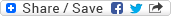## Friday, May 23, 2008

### Rope Bridge, Torch, Four People - Puzzle

Puzzle: Four people need to cross a rope bridge, which is strong enough to support only two people at a time. First person takes 1 minute to cross the bridge, Second person takes 2 minutes, Third person takes 5 minutes, and the Fourth person takes 10 minutes to cross the bridge. They have a torch which has battery left only for 17 minutes and the bridge can not be crossed without light. How will they manage to cross the bridge?

Solution: Let's say the four people as P, Q, R, and S. Based on the info we have, let's consider P takes 1 minute, Q takes 2 minutes, R takes 5 minutes, and S takes 10 minutes to cross the bridge.

Now, they can manage to cross the bridge in time if they follow the below steps:-
• Step #1: P and Q cross the river. Total time taken so far = 2 minutes
• Step #2: Q comes back. Total time taken so far = 2 + 2 = 4 minutes
• Step #3: R and S cross the river. Total time takes so far = 4 + 10 = 14 minutes
• Step #4: P comes back (he's at the other end). Total time so far = 14 + 1 = 15 minutes
• Step #5: P & Q cross the river. Total time taken so far = 15 + 2 = 17 minutes

We can have another valid solution similar to the above in case P comes back instead of Q in Step #2:-
• Step #1: P and Q cross the river. Total time = 2 minutes
• Step #2: P comes back. Total time = 2 + 1 = 3 minutes
• Step #3: R and S cross the river. Total time = 3 + 10 = 13 minutes
• Step #4: Q comes back. Total time = 13 + 2 = 15 minutes
• Step #5: P and Q cross the river. Total time = 15 + 2 = 17 minutes